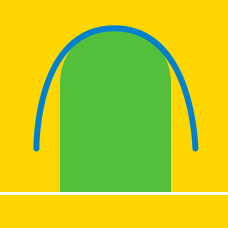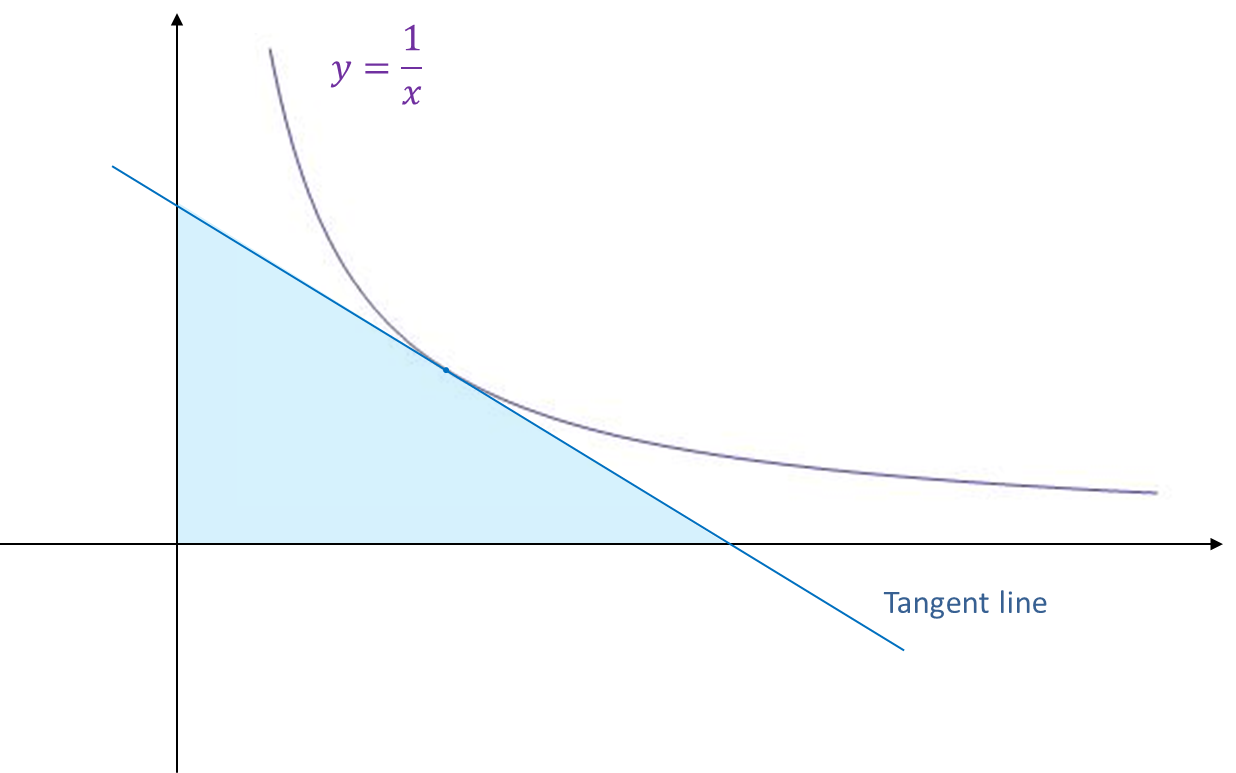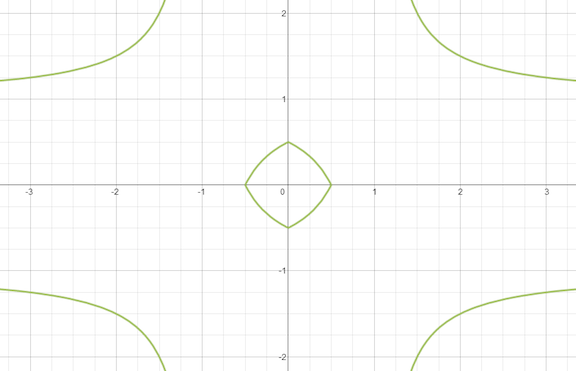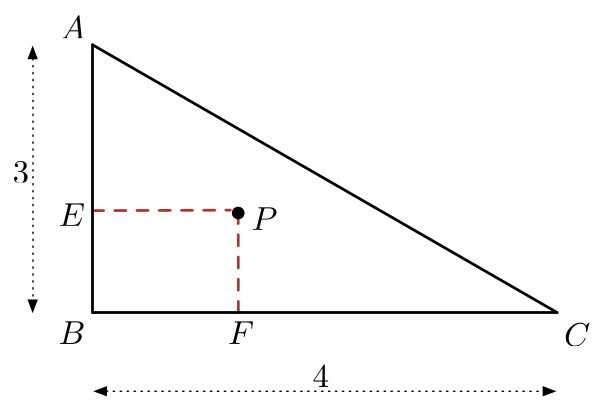Calculus

# Area Between Curves: Level 3 ChallengesThe area of triangles enclosed by the axis and the tangent to $y=\dfrac1x$ is always constant, no matter what $x$ you pick. Find the value of this area.Above is the graph of the equation $|x|+|y|-|x||y|=0.5$ Let the area of the cute little pillowed diamond in the middle be $A$. What is the value of $\lfloor 1000A\rfloor$?

Find the area bounded by the curve $f(x)=x+\sin(x)$ and its inverse function between the values $x=0$ and $x=2\pi$.

A square has vertices at $(1,1), (-1,1), (-1,-1), \text{ and } (1,-1).$

Let $S$ be the region of all points inside the square which are nearer to the origin than to any edge. What is the area of $S$?$\triangle ABC$ is constructed such that $AB=3$, $BC=4$, and $\angle ABC=90^{\circ}$. Point $P$ is chosen inside $\triangle ABC$, and points $E$ and $F$ are drawn such that they form line segments with $P$ that are perpendicular to sides $AB$ and $BC$, respectively. If $\mathbf{L}$ is the locus of all points $P$ such that $[PEBF]\ge 1$, then find the value of $\left\lfloor 1000\dfrac{[\mathbf{L}]}{[ABC]}\right\rfloor$

$\text{Details and Assumptions:}$

$[\mathbf{N}]$ means the area of the locus $\mathbf{N}$, and $[PQRS]$ means the area of $PQRS$. $\lfloor x \rfloor$ is the floor function.

×